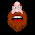## Tuesday, April 19, 2016

### Sound sensor Arduino

Tutorial on how to interface sound sensor with Arduino. More precisely, Sound Sensor. I will show how to detect ambient sound and how to handle the signal generated by this module. This board along with the microphone, has a small built-in amplifier (integrated circuit LM386), because only the microphone would not be able to send data for Arduino. The connection scheme is very clean, composed of only 3 pins: Vcc, GND and S (signal). In the middle of the plate, there is a potentiometer for sensitivity adjustment.
The board works with 5V voltage, and the signal pin should be connected preferably to an analog port of Arduino, since the generated signal is variable, and thus we can see the different levels of noise picked up by the microphone. The circuit tests will consist of the module Sound Sensor , plus the display 16x2 LCD , which we've covered here on the site, in this article . If you do not have this display, do not worry because the program is easily adaptable to other models of LCD displays . The display at its top, will show the sound level (Low, Medium and High), and bottom , a bar that will follow in real time the sound level detected by the microphone will be shown:

The program does not use any own library for the sound sensor module, as it will only make the reading of the variable signal received by the Arduino analog port. For the LCD display is used to already known LiquidCrystal .To improve the program's accuracy, I chose to read the sign 128 times using the NUM_Measure variable. Then calculate the mean value and use this value to generate the LCD sound level indication. The graph on the display bottom line uses the signal information in real time. If you choose not to use the display, the data is also shown in the Serial Monitor.

```// Program: Noise Levels with Sound Sensor

int num_Measure = 128 ; // Set the number of measurements
int pinSignal = A0; // pin connected to pin O module sound sensor
long Sound_signal;    // Store the value read Sound Sensor
long sum = 0 ; // Store the total value of n measurements
long level = 0 ; // Store the average value
int mostranivel = 0 ; // Used to generate the graph below
int delete = 0 ; // Variable assist to generate the graph below

#include <LiquidCrystal.h> // Load the LCD library

// Set the pins that will be connected to the LCD
LiquidCrystal LCD ( 12 , 11 , 5 , 4 , 3 , 2 );

// Array to mount the symbol graphic
byte [ 8 ] =
{B10000, B11000, B11100, B11110, B11110, B11100, B11000, B10000,};

void setup ()
{
pinMode (pinSignal, INPUT); // Set the signal pin as input
Serial.begin ( 9600 );
lcd.begin ( 16 , 2 ); // Initialize LCD
lcd.clear ();    // Clear the LCD
lcd.setCursor ( 0 , 0 ); // Shifts the column 0, row 0
lcd.print ( "Level : " );
lcd.createChar ( 1 , a); // Assigns the "1" the value of the array "A",
// drawing the symbol graph
}

void loop ()
{
// Performs 128 signal readings
for ( int i = 0 ; i <num_Measure; i ++)
{
sum  = sum + Sound_signal;
}

level = sum / num_Measure; // Calculate the average value
// Convert the read value to a value between 0 and 15
mostranivel = map (signal, 0 , 500 , 0 , 15 );

// Generate the graph below
for ( int i = 0 ; i <mostranivel; i ++)
{
lcd.setCursor (i, 1 );
lcd.write ( 1 );
erases = 15 - i;
for ( int x = i; x < 15 ; x ++)
{
lcd.setCursor (x + 1 , 1 );
lcd.write ( "" );
}
}

// Check the signal level and displays on the screen
if (level> 0 && level < 100 )
{
lcd.setCursor ( 8 , 0 );
lcd.print ( "Low" );
Serial.print ( "Low Level" );
Serial.print ( "- Media" );
Serial.println (level);
}

if (level> 100 && level < 200 )
{
lcd.setCursor ( 8 , 0 );
lcd.print ( "Medium" );
Serial.print ( "Medium Level" );  ```
```    Serial.print ( "- Media" );
Serial.println (level);
}

if (level> 200 )
{
lcd.setCursor ( 8 , 0 );
lcd.print ( "High" );
Serial.print ( "High level" );
Serial.print ( "- Media" );
Serial.println (level);
}

sum = 0 ; // Reset the sum of the measurement values
}
```

You can adjust the level by changing set points. observe the output also on serial terminal

#### 1 comment:

1.unqualified-id before 'delete'